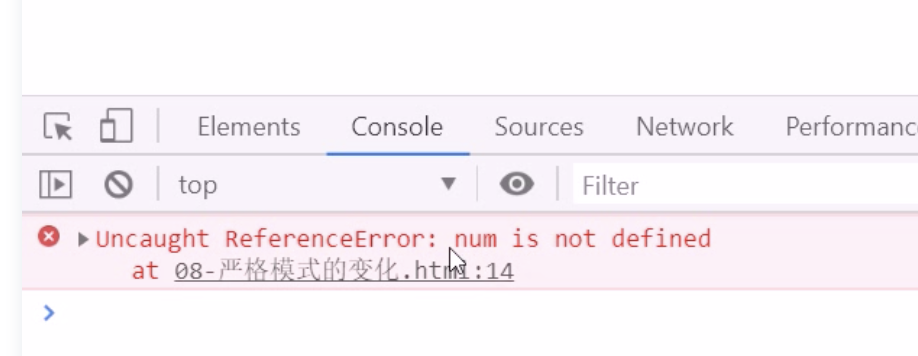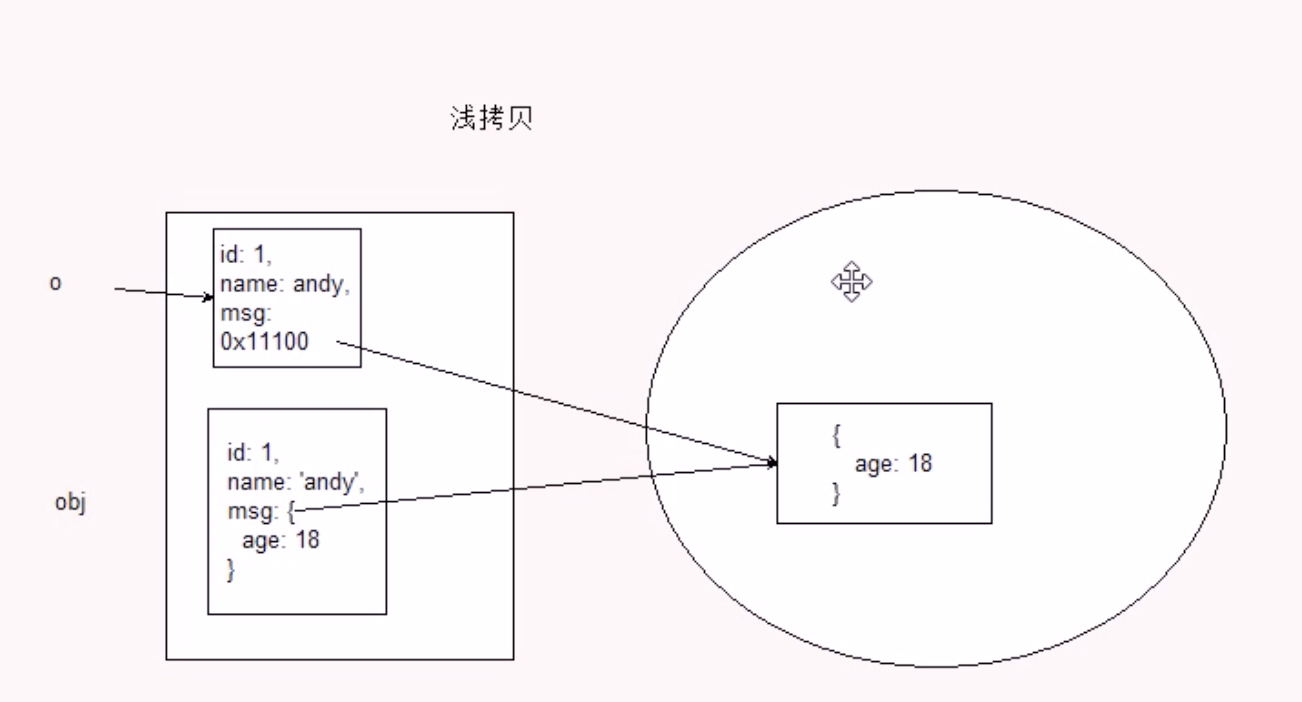## Take you to learn the advanced function of JavaScript (2)

Tell me Zhan to hide 2020-11-06 20:37:31

Last article shared 《 Take you to study Javascript Advanced function in the （ One ）》, Continue today javascript The content of advanced function of .

### 1 Strict mode

#### 1.1 What is the strict pattern

JavaScript In addition to providing normal mode , It also provides a rigorous model （strict mode）.ES5 The strict model is to adopt a restrictive Javascript A way of variation . I.e. operate under strict conditions js Code .
The strict pattern is in IE10 Only in the above version of the browser will be supported , Older browsers will be ignored .
Strict mode for normal javascript Some semantic changes have been made ：

1. Eliminated Javascrip Some unreasonable grammar 、 Not rigorous , Reduced some weird behavior .
2. Eliminates some of the unsafe aspects of code running , Ensure the safety of code operation .
3. Improve compiler efficiency , Increase running speed .
4. Disabled in ECMAScript Some of the syntax that may be defined in future versions of , For future new versions of Javascript Pave the way . Than

Such as some reserved words ：class,enum,export,extends,import,super You can't make variables .

#### 1.2 Turn on strict mode

Strict patterns can be applied to entire scripts or individual functions . So in use , We can divide strict mode into script on strict mode and
Turn on strict mode for the function in two cases

1. Turn on strict mode for the entire script

Turn on strict mode for the entire footstep file , You need to make a specific statement before all statements "use strict"

``````<scirpt>
"use strict"
console.log(" This is the most rigorous model ")
</scirpt>``````
1. Turn on strict mode for functions

To turn on strict mode for a function , Need to put "use strict";（ or ’use strict’） The declaration is placed before all statements in the body of the function .

``````<script>
function fn() {

'use strict' // The following code follows a strict pattern
}
</script>``````

#### 3.3 Changes in strict patterns

The strict pattern is right javascript The language of
Law and action , There have been some changes

1. Variables specify
• In normal mode , If a variable is not declared, it is assigned a value , The default is global variables .
• var A statement of order , And then use
``````<script>
‘use strict’
num = 10
console.log(num)
</script>``````

The effect is as followsIt is strictly forbidden to delete declared variables .

1. In strict mode this Point to the problem
• Previously in global scope functions this Point to window object .
• In strict mode, in the global scope of the function this things undefined.
• Before the constructor without new You can also call , When a normal function ,this Point to global object .
• In strict mode , If the constructor does not add new call ,this Will report a mistake . new The instantiated constructor points to the created object instance .
• In strict mode , In the timer this Point to or window.
• In strict mode , event 、 The object still points to the caller .
1. Function change
• In strict mode, functions cannot have duplicate parameters
• Functions must be declared at the top level , The new version of the js Will introduce “ Block level scope ”（ES6 Has introduced ）. In order to be in line with the new version , Functions are not allowed to be declared within blocks of code that are not functions , If in if,for Statement to declare a function .

### 2 Higher order function

Higher order functions are functions that operate on other functions , It takes a function as a parameter or a function as a return value .

``````function fn(callback) {

callback && callback()
}
fn(function(){

})``````
``````function fn() {

return function() {

}
}
fn()``````

here fn It's a higher-order function
Function is also a data type , It can also be used as a parameter , Pass to another parameter to use , The most typical is as a callback function

### 3 Closure

#### 3.1 Variable scope

Variables are divided into two types according to their scopes ： Global and local variables .

1. Global variables can be used inside functions
2. Local variables cannot be used outside a function
3. When the function is finished , Local variables in this scope will be destroyed .

#### 3.2 What is a closure

A closure is a function that has access to a variable in the scope of another function . in other words , A scope can access local variables inside another function .

``````//fn External scopes can access fn Internal local variables
function fn() {

var num = 10
function fun () {

console.log(num) // You can visit num
}
return fun
}
var f = fn()
f() //10``````
``````//fn External scopes can access fn Internal local variables
function fn() {

var num = 10
// Returns an anonymous function
return function() {

console.log(num) // You can visit num
}
}
var f = fn()
f() //10``````

The main function of closures is ： It extends the scope of variables

#### 3.3 Closure cases

1. Cycle registration click events
``````//html
<ul class="nav">
<li>a</li>
<li>b</li>
<li>c</li>
<li>d</li>
</ul>
//js
// Click on li Output current li The index of
// Using dynamic track and field attributes
var lis = document.querySelector('.nav').querySelectorAll('li')
for(var i=0 ;i<lis.length; i++) {

lis.index = i
lis[i].onclick = function() {

console.log(this.index)
}
}
// Using closures
var lis = document.querySelector('.nav').querySelectorAll('li')
for(var i=0 ;i<lis.length; i++) {

(function(i) {

lis[i].onclick = function() {

console.log(i)
}
})(i)
}``````
1. In the loop setTimeout()
``````//html
<ul class="nav">
<li>a</li>
<li>b</li>
<li>c</li>
<li>d</li>
</ul>
//js
// Use closures
var lis = document.querySelector('.nav').querySelectorAll('li')
for(var i=0 ;i<lis.length; i++) {

(function(i){

setTimeout(function() {

console.log(lis[i].innerHTML)
},3000)
})(i)
}``````
1. Calculate the taxi price
``````var car = （function() {

var start = 13;
var total = 0;
return {

// Normal price
price: function(n) {

if(n <= 3) {

total = start
} else {

total = start + (n-3)* 5
}
},
// After the congestion
yd: function(flag) {

flag? total+ 10 :total
}
}
}）()
console.log(car.price(5))
console.log(car.yd(true))``````

#### 3.4 Closure summary

1. What is a closure ？

Closure is a function （ One scope can access the local variables of another function ）

1. What do closures do ？

Extend the scope of the variable

### 4 recursive

#### 4.1 What is recursion

If a function can call itself internally , So this function is recursive .
Recursive functions work just as well as loops
Because recursion is easy to happen “ Stack overflow ”(stack overflow), So you have to add exit conditions return

#### 4.2 Using recursion to solve mathematical problems

1. seek 123…*n
``````function fn(n) {

if(n = 1) {

return 1
}
return n * fn(n-1)
}
console.log(fn(3)) // 6``````

#### 4.3 Use recursion to find ： according to id Return the corresponding data object

``````var data = [{

id:1,
name:' Home appliance ',
goods: [
{

id: 11,
gname: ' The refrigerator '
},
{

id: 12,
gname: ' Washing machine '
}
]
},
{

id: 2,
name: ' dress '
}
]
function getObj(arr, id) {

var o = {
}
arr.forEach(function(item){

if(item.id === id) {

o= item
} else if(item.goods && item.goods.length>0) {

o =getObj(item.goods, id);
}
})
return o
}
console.log(getObj(data, 1))``````

### 5 recursive

#### 5.1 Shallow copy and deep copy

1. Shallow copy is just a layer of copy , Deeper object level copy only references .
2. Deep copy copy copy multilayer , Every level of data is copied .
3. Object.assign(target, …sources) es6 New method can be shallow copy
``````var obj = {

id: 1,
name: 'andy',
msg: {

age: 18
}
}
var o = {
}
for(var k in obj) {

// k It's the property name
o[k] = obj[k]
}
console.log(o)
o.msg.age = 20
console.log(obj) //obj change
// use es6 Grammatical sugar
Object.assign(o, obj)````````````// Deep copy , Using recursion
function deepCopy(newObj, oldObj) {

for(var k in oldObj) {

// Determine what type of attribute value we have
var item = oldObj[k]
// Determine if it's an array
if(item instanceof Array) {

newObj[k] = []
deepCopy(newObj[k], item)
} else if(item instanceof Object) {

// Judge whether it is the object
newObj[k] = {
}
deepCopy(newObj[k], item)
} else {
// It's a simple data type
newObj[k] = item
}
}
}
deepCopy(o,obj)
console.log(o)``````

### summary

This article mainly shares the strict pattern of functions 、 Higher order function 、 Closure 、 recursive 、 Deep copy 、 The usage and application of knowledge points such as shallow copy .